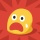Home
IT Knowledge
Inspiration
Languages

# React - useState set method does not reflecting my change immediately

5 pointsJun-L
413

Hi there!

What is the problem with my component? When I try to set state, and use it again the state doesn't reflect previous change. What is wrong with my code?

``````const Calculator = ({a, b, operator}) => {
const [result, setResult] = useState();
useEffect(() => {
if (operator === '+') {
setResult(a + b)
}
console.log('Result: ' + result); // <------ logs wrong value
}, [a, b, operator]);
return (
<div>Result: {result}</div>
);
};``````

Why component dysplays proper result on my site, and console logs wrong result value?

7 pointsJun-L
413

`useState` setter schedules update action that will be visible in the next rendering cycle.

`useState` set operation is `asynchronous` and will not be reflected immediately.

You can try to use the following source code to see the result change effect:

``````const Calculator = ({a, b, operator}) => {
const [result, setResult] = useState();
useEffect(() => {
if (operator === '+') {
setResult(a + b)
}
}, [a, b, operator]);
useEffect(() => {
console.log('Result: ' + result); // <------ solution for wrong value in question code
}, [result]);
return (
<div>Result: {result}</div>
);
};``````

second `useEffect()` will be triggered always on `result` changed, and after the component will be mount/ready.

## Alternative solutions

1. Helper `newResult` variable/constant:

``````const Calculator = ({a, b, operator}) => {
const [result, setResult] = useState();
useEffect(() => {
if (operator === '+') {
const newResult = a + b;
setResult(newResult);
console.log('Result: ' + result); // <------ solution for wrong value in question code
}
}, [a, b, operator]);
return (
<div>Result: {result}</div>
);
};``````

1. Setter with callback:

``````const Calculator = ({a, b, operator}) => {
const [result, setResult] = useState();
useEffect(() => {
if (operator === '+') {
setResult(result => {
const newResult = a + b;
console.log('Result: ' + result); // <------ solution for wrong value in question code
return newResult;
});
}
}, [a, b, operator]);
return (
<div>Result: {result}</div>
);
};``````